# What is a PCB Transmission Line?

by | Feb 3, 2020 | 7 comments

A PCB transmission line is a type of interconnection used for moving signals from their transmitters to their receivers on a printed circuit board. A PCB transmission line is composed of two conductors: a signal trace and a return path which is usually a ground plane. The volume between the two conductors is made up of the PCB dielectric material.

The alternating current that runs on a transmission line usually has a high enough frequency to manifest its wave propagation nature. The key aspect of the wave propagation of the electrical signals over a transmission line is that the line has an impedance at every point along its length and if the line geometry is the same along the length, the line impedance is uniform. We call such a line a controlled impedance line. Non-uniform impedance causes signal reflections and distortion. It means that at high frequencies, transmission lines need to have a controlled impedance to predict the behavior of the signals. Read our post: why controlled impedance really matters?

It is crucial to not ignore the transmission line effects in order to avoid signal reflections, crosstalk, electromagnetic noise and other issues which could severely impact the signal quality and cause errors.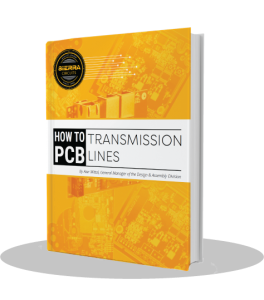## What are the types of transmission lines?

There are usually two basic types of signal transmission line interconnects used in PCBs: microstrips and striplines. There is a third type – coplanar without a reference plane but it is not very common in use.

A microstrip transmission line is composed of a single uniform trace – for the signal – located on the outer layer of a PCB, and parallel to a conducting ground plane, which provides the return path for the signal. The trace and the ground plane are separated by a certain height of PCB dielectric. Below is an uncoated microstrip: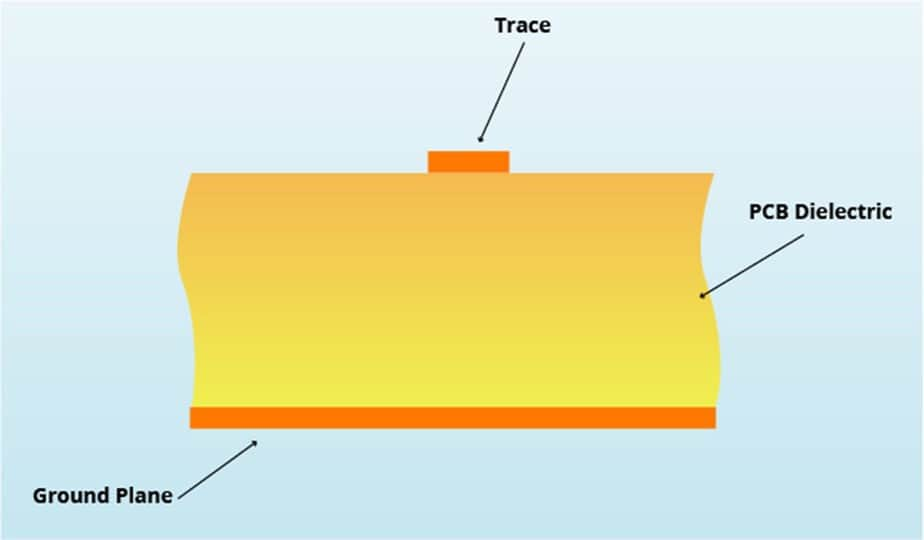A stripline is composed of a uniform trace – for the signal – located on the inner layer of a PCB. The trace is separated on each side by a parallel PCB dielectric layer and then a conducting plane. So it has two return paths – reference plane 1 and reference plane 2.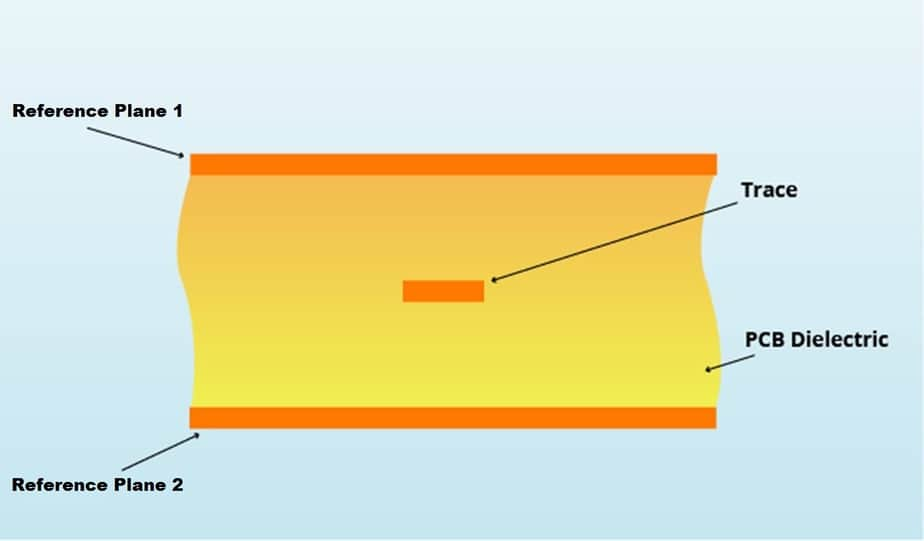In addition to conventional microstrips and striplines described above, a coplanar waveguide structure has the signal trace and the return path conductor on the same layer of the PCB. The signal trace is at the center and is surrounded by the two adjacent outer ground planes; it is called “coplanar” because these three flat structures are on the same plane. The PCB dielectric is located underneath. Both microstrips and striplines may have a coplanar structure. Below is a coplane microstrip waveguide with a ground plane: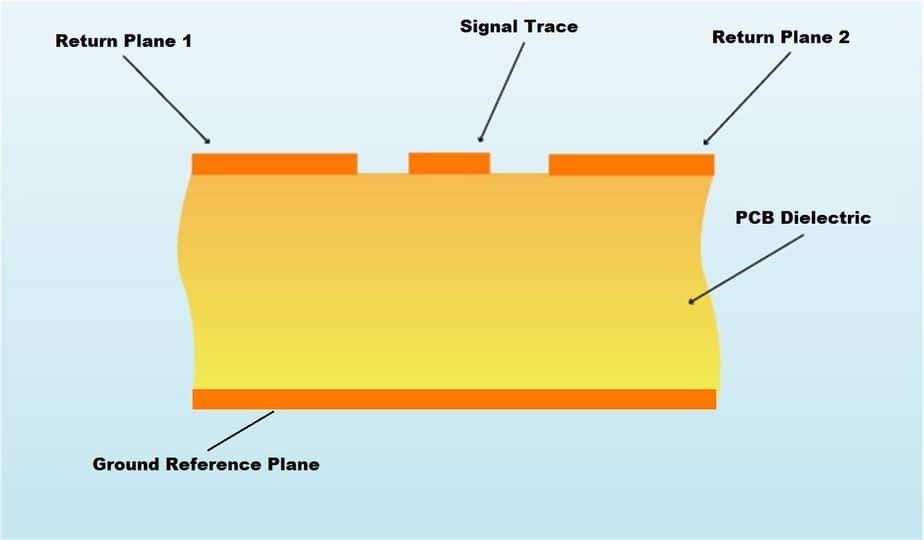### Example of a coaxial cable (which is not a PCB transmission line):

A coaxial line has a circular shape and is not a PCB transmission line. This circular cable is composed of a central wire conductor for the signal and an outer circular conductor for the return path. The space between the two conductors is filled by a dielectric material. The outer conductor wire completely surrounds the signal wire. Coaxial lines are mostly used as cables for high-frequency applications, such as television, etc. A coaxial cable must have a uniform geometry of conductors and the properties of the dielectric material must be uniform along the entire geometry.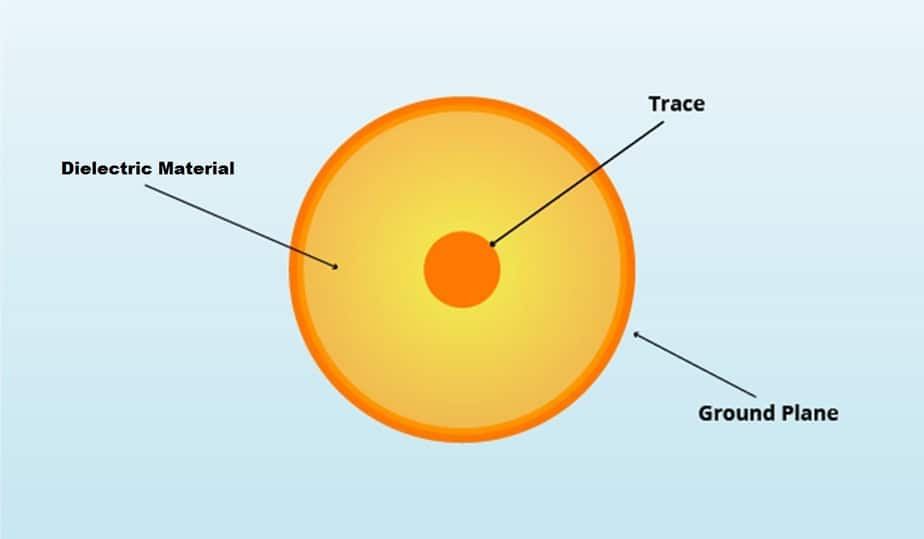It is essential to keep in mind that a PCB transmission line is composed of not only the signal trace but also the return path, which is usually an adjoining ground plane or a coplanar conductor, or a combination of both.

### When is an interconnection to be treated as a transmission line?

The set of electrical conductors (as stated above, at least two conductors are required: one for the signal and the other one for the return path, which is usually a ground plane) used for connecting a signal between its source and its destination is called a transmission line (and not just an interconnection) if it is not possible to ignore the time it takes for the signal to travel from the source to the destination, as compared to the time period of one-fourth of the wavelength of the higher frequency component in the signal.

Two very important properties of a transmission line are its characteristic impedance and its propagation delay per unit length; and if the impedance is not controlled along its entire length, or the line is not terminated by the right value of impedance, signal reflections, crosstalk, electromagnetic noise, etc. will occur, and degradation in signal quality may be severe enough to create errors in information being transmitted and received. Read our post on understanding signal integrity in PCBs.

When the signal frequencies (in case of analog signals) or the data transfer rates (in case of digital signals) are low (less than 50 MHz or 20 Mbps), the time it will take for a signal to travel from its source to its destination on a PCB would be very small (< 10%) compared to the time period of one-fourth of a wavelength or the fastest rise time of a digital pulse signal. In this case, it is possible to approximate the interconnect by assuming that the signal at the destination follows the signal at its source at the same time. In such a low-speed scenario, the PCB signal can be analyzed by conventional network analysis techniques and we can ignore any signal propagation time or transmission line reflections, etc.

However, when dealing with signals at higher frequencies or higher data transfer rates, the signal propagation time on PCB conductors between the source and the destination cannot be ignored in comparison to the time period of one-fourth of a wavelength or the fastest pulse rise time. Therefore, it is not possible to analyze the behavior of such high-speed signals on PCB interconnects using ordinary network analysis techniques. The interconnects need to be considered as transmission lines and analyzed accordingly.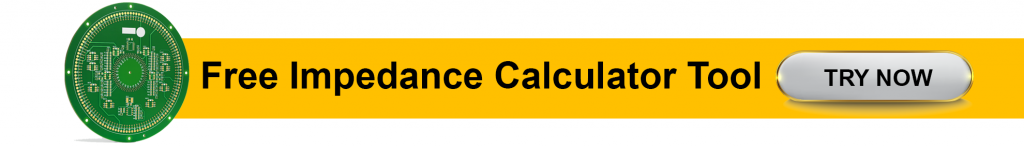## How to predict the signal behavior on microstrip and stripline?

At high frequencies, transmission lines need to have a controlled impedance to predict the behavior of the signals and avoid signal reflections, crosstalk, electromagnetic noise, etc. which could damage the signal quality and cause errors. Read our post on controlled impedance routing using Altium.

This is the reason why you need to know at which speed signals propagate on transmission lines and the time they take to do so. We will give you a few equations to calculate the signal speed and the propagation delay for both striplines and microstrips.

### What is signal speed?

Let’s first discuss the speeds at which signals propagate on a PCB interconnect.

Electromagnetic signals travel in vacuum or air at the same speed as of light, which is: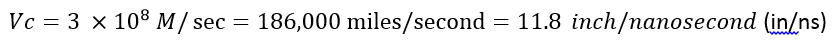A signal travels on a PCB transmission line at a slower speed, affected by the dielectric constant (Er) of the PCB material. The relations for calculating the signal speed on a PCB are given below: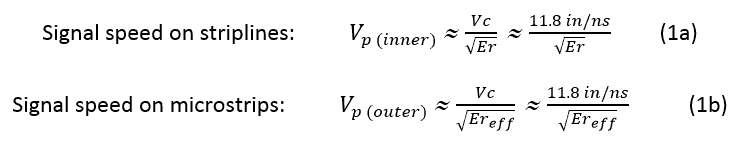Where:
Vc is the velocity of light in vacuum or air
Er is the dielectric constant of the PCB material
Ereff is the effective dielectric constant for microstrips; its value lies between 1 and Er, and is approximately given by: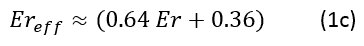Thus, the speeds of signals on a PCB is less than that in air. If Er ≈ 4 (like for FR4 material types), then the speed of signals on a stripline is half that in air, i.e. it is about 6 in/ns.

Henceforth, we can use Vp to denote the speed of signals on a PCB.### What is propagation delay?

The propagation delay is the time taken by a signal to propagate over a unit length of the transmission line: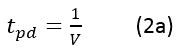Where:
V is the signal speed in the transmission line

In vacuum or air, it equals 85 picoseconds/inch (ps/in).

On PCB transmission lines, the propagation delay is given by: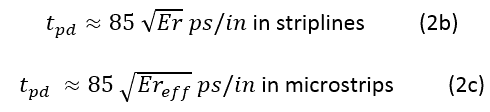### What materials are used in PCB transmission lines?

The most used materials are Isola 370HR, Isola I-Speed, Isola I-Meta, Isola Astra MT77, Tachyon 100G, Rogers 3003, and the Rogers 4000 series.

The signal speeds and propagation delays for a few PCB materials are given in the table below: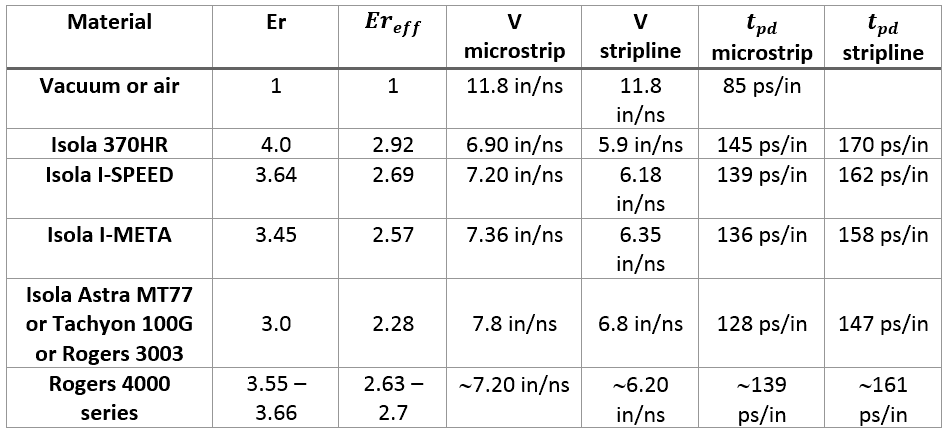## What are the transmission line effects?

### Let’s discuss critical length, controlled impedance and rise/fall time.

As we mentioned above, for high-speed or high-frequency signals, we need to consider the transmission line effects. We can use a few thumb rules:

In case of high-frequency analog signals, let the maximum frequency content in the signal =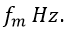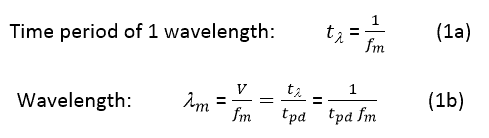### How to define the critical length?

For analog signals, the critical length lc is defined as one-fourth of the wavelength of the highest signal frequency contained in the signal.In case of digital signals, the fastest rise/fall time of a signal pulse is the most important parameter. It defines the transition time from one logic level to another logic level. Basically, the transition time of the data bit. For digital signals, the critical length lc is defined as the line length over which the signal propagation time is half of the fastest rise/fall time of the signal pulses.

If tr = fastest rise/fall time of the digital signal, the time of the propagation of the signal over the length lc is tpd (propagation delay). lc = tr/2 (by definition of lc).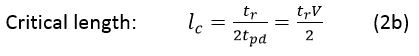This definition implies that the signal should be able to travel from the source over a length lc of the line. And then return over the same length lc of the line back to the source point in a total time equal to the rise/fall time tr.

Equations 2a and 2b above are related if we consider the highest frequency content in a digital signal rise/fall time.

The highest frequency content in a digital signal of rise/fall time tr is given by (as per Fourier Analysis):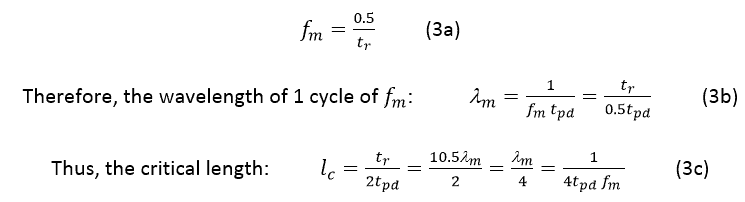Which is the same as Equation 2a above.

### What is a shortline?

If the line length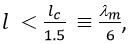there is a shortline and it is not necessary to consider its transmission line effects, nor to design it as a controlled impedance line.

But if the line length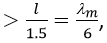it then becomes necessary to consider the transmission line effects and to design such lines as controlled impedance lines.

Example:

If the fastest signal rise/fall time is: tr = 1ns, then, assuming the FR4 material with a dielectric constant Er = 4,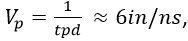and the critical length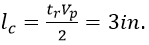Therefore, signal traces longer than 3/1.5 = 2 inches need to be designed as controlled impedance lines. Note here that tr of 1ns corresponds to a maximal signal frequency: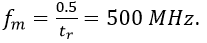### How to estimate the rise/fall time from data transfer rate (DTR) or clock frequency?

The data transfer rate (DTR) is measured in bits per second (bps or bits/sec or b/s). And the clock frequency (Fclock) in Hz.

DTR is usually ≥ 2 Fclock. Henceforth, it will be safe to use the following rule: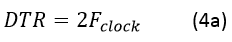If we don’t know the signal rise/fall time, we can use the following rule: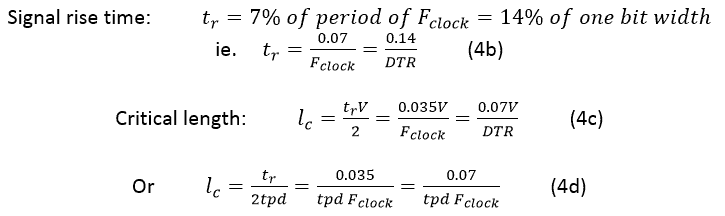Example:

For Fclock = 1GHz or DTR = Gbps, and for a PCB material with Er = 4, we get: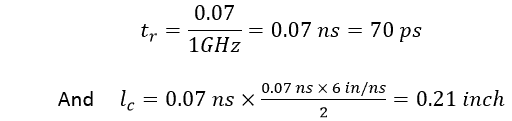### How to obtain the 3 dB bandwidth?

For a signal with rise/fall time tr, the 3 dB bandwidth is given by the following rule: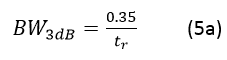Therefore, for a clock of frequency Fclock, we get: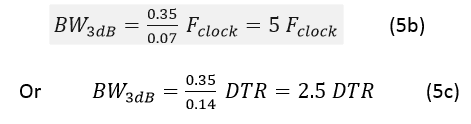## How to analyze a PCB transmission line?

Basically, high-speed or high-frequency signals generate electromagnetic fields around them during their travel along a transmission line, and their behavior is best analyzed using Maxwell’s electromagnetic equations and the theory of electromagnetic wave propagation. In this method, we have to deal with electric and magnetic fields instead of the usual voltages and currents. The voltage between the signal line and its return path will generate an electric field and a current in the conductors, which in turn will create a magnetic field around them. Thus, voltage, current, and electric and magnetic fields all travel as waves along the transmission line.

There exists a close analogy between the electromagnetic waves propagation and the propagation of voltages and currents along a transmission line. As it is easier to think in terms of familiar voltages and currents rather than electric and magnetic fields, we should use the voltage/current analysis of the transmission line in the following treatment.

### What are the transmission line parameters?

A transmission line is a large number of infinitesimal length segments, and each segment can be analyzed by network theory concepts at a particular point in space and time, ignoring the travel time in the infinitesimal segment as its length is extremely small.

In this analysis technique, we will be dealing with quantities like voltages and currents, and transmission line parameters like resistance, inductance, capacitance, and conductance. We will model an infinitesimal segment of a PCB transmission line in terms of the following parameters of the transmission line:

R = the resistance of the transmission line per unit length (or p.u.l.) (R in Ohms p.u.l.)
L = the inductance of the transmission line p.u.l. (L in Henrys p.u.l.)
C = the capacitance of the transmission line p.u.l. (C in Farads p.u.l.)
G = the conductance of the transmission line p.u.l. (G in Mhos p.u.l.)

We can denote the length of the infinitesimal transmission line by Δx.

Then we can picture this transmission line segment as follows:Where:
V(x,t) = signal voltage at location x at time t
I(x,t) = signal current at location x at time t

Let’s do the analysis of this circuit in the frequency domain. Here, we assume that the signals vary with time sinusoidally with an angular frequency ω, so that the time-varying part of V(x,t) and I(x,t) can be shown by the factor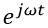and we now have: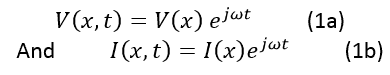Using Kirchhoff’s laws on the above segment, we obtain the following relations: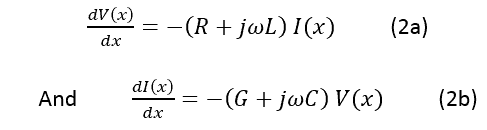And from these, by differentiation, we get: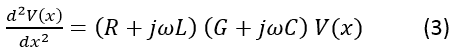A solution of this equation is: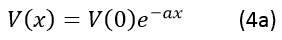And from Equation 2,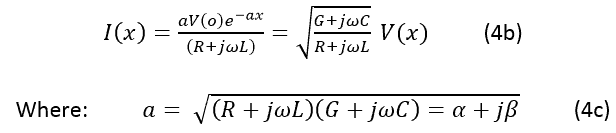α has units of p.u.l.

If we multiply Equation 4a byto reincorporate the time variations of the sinusoidal voltages and currents, we will see that now the equations represent voltage and current signal waves traveling in the positive x direction over the transmission line: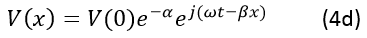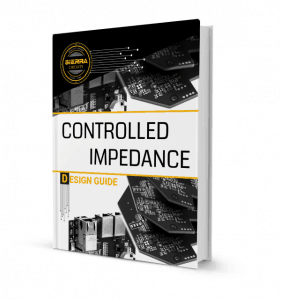1.The transition to transmission line behavior with digital signals has nothing to do with the data transfer rate. It is actually related to the signal rise time. You pointed this out correctly in a later article in this series.

•Hello Zachariah, The data transfer rate defines the pulse width of the digital signals. The pulse width’s finite duration imposes a constraint on the maximum value of the pulse edge rise and fall times.

The higher the transfer rate, the lesser will be the required rise time. Thus, the data transfer rate is related to the rise time. From a given data transfer rate, it is possible to calculate the approximate rise time. This is the thought process that led us to say that the data transfer rate also impacts transmission line behavior. Thank You!

2.In equation 2b you assume the voltage across the GC branch is V(x), but it is more precisely V(x+dx). It seems to change the whole solution, although the final form of the formula is well known as the telegraph equation. The only explanation I can find is there is an assumption that dVx is negligible. Is that so?

•Hello Yaniv, In the blog, in the interest of not using too much space, I did not show the entire mathematical limiting process that one uses in deriving the derivatives of functions in detail.

How 2(b) takes its final form as a differential equation is explained below:
The current in the GC branch is given by (using Kirchhoffs law):

I(x) – I(x+Δx) = ( G Δx + jwC Δx ) V(x+Δx)
Dividing both sides by Δx and taking limits as Δx approaches zero, we get the equation 2(b):
– dI(x)/dx = (G+jwC) V(x).

I hope that clarifies it. Thank You!

3.Hello, There are questions

FR-4:4Er Fm: 100MHz DTR: 200Mbps

Vc 11.8 inch/ns > 299.72 mm/ns
V 0.0847 inch/ns > 0.00334 mm/ns
84.7 ps/mm > 3.34 ps/mm
Srtripline (Vp) 5.9 inch/ns 149.86 mm/ns
Srtripline (tPD) 0.2 ps/inch 0.006673 ns/mm
t(periode) 10 ns

Wavelength(λm) 1498.6 mm = Vp / Fm
1498.6 mm = t / tpd
1498.6 mm = 1 / tpd * Fm

Analog Ctrical Length 374.65 mm = λm / 4
374.65 mm = 1 / 4 * tpd * Fm

tr (time rise) 5ns = 0.5 / Fm

λm = Wavelength 1498.6 mm = 1 / Fm * tpd
1498.6 mm = tr / 0.5 * tpd

Critical Length(lc): Anlog = Digital 374.65 mm = tr / 2 * tpd
374.65 mm = 0.5 * λm / 2
374.65 mm = 1 / 4 * tpd * Fm

Trace line < 277.5185185 mm = lc / 1.5
277.5185185 mm = λm / 5.4
≡ 249.7666667 mm = λm / 6

Is the above solution process correct?

•